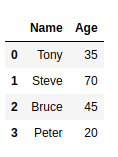How to Convert Pandas DataFrame into a List?

• Last Updated : 03 May, 2021

Let’s discuss how to convert Pandas dataframe to List. First, let’s create a Basic DataFrame:

Python3

 import pandas as pd    # Creating a dictionary to store data data = {'Name':['Tony', 'Steve', 'Bruce', 'Peter' ],         'Age': [35, 70, 45, 20] }    # Creating DataFrame  df = pd.DataFrame(data)    # Print the dataframe df

Output :At times, you may need to convert your pandas dataframe to List. To accomplish this task, ‘ tolist() ‘ function can be used. Below is a basic example to use this function and convert the required DataFrame into a List.

Python3

 df.values.tolist()

Output :

[['Tony', 35], ['Steve', 70], ['Bruce', 45], ['Peter', 20]]

Here, Each inner list contains all the columns of a particular row.

Pandas DataFrame can be converted into lists in multiple ways. Let’s have a look at different ways of converting a DataFrame one by one.

Method #1: Converting a DataFrame to List containing all the rows of a particular column:

Python3

 import pandas as pd    # Creating a dictionary to store data data = {'Name':['Tony', 'Steve', 'Bruce', 'Peter' ] ,         'Age': [35, 70, 45, 20] }    # Creating DataFrame  df = pd.DataFrame(data)    # Converting DataFrame to a list containing # all the rows of column 'Name' names = df['Name'].tolist()   # Printing the converted list. print(names)

Output:

['Tony', 'Steve', 'Bruce', 'Peter']

Method #2: Converting a DataFrame to Nested List containing all the rows of all the columns:

Python3

 import pandas as pd    # Creating a dictionary to store data data = {'Name':['Tony', 'Steve', 'Bruce', 'Peter' ] ,         'Age': [35, 70, 45, 20] }    # Creating DataFrame df = pd.DataFrame(data)   # Creating an empty list res=[]   # Iterating through the columns of # dataframe for column in df.columns:           # Storing the rows of a column     # into a temporary list     li = df[column].tolist()           # appending the temporary list     res.append(li)       # Printing the final list print(res)

Output:

[['Tony', 'Steve', 'Bruce', 'Peter'], [35, 70, 45, 20]]

Method #3: Converting a DataFrame to a list that contains lists having all the columns of a row.

Python3

 import pandas as pd    # Creating a dictionary to store data data = {'Name':['Tony', 'Steve', 'Bruce', 'Peter' ] ,         'Age': [35, 70, 45, 20] }    # Creating DataFrame df = pd.DataFrame(data)    # Converting dataframe to list li = df.values.tolist()   # Printing list print(li)

Output :

[['Tony', 35], ['Steve', 70], ['Bruce', 45], ['Peter', 20]]

Method #4: Converting a DataFrame to a list that contains lists having all the columns of a row along with column names.

Python3

 import pandas as pd    # Creating a dictionary to store data data = {'Name':['Tony', 'Steve', 'Bruce', 'Peter' ] ,         'Age': [35, 70, 45, 20] }    # Creating DataFrame df = pd.DataFrame(data)    # Converting dataframe to list li = [df.columns.values.tolist()] + df.values.tolist()   # Printing list print(li)

Output:

[[‘Name’, ‘Age’], [‘Tony’, 35], [‘Steve’, 70], [‘Bruce’, 45], [‘Peter’, 20]]

My Personal Notes arrow_drop_up
Recommended Articles
Page :